×#### Thank you for registering.

One of our academic counsellors will contact you within 1 working day.

Click to Chat

1800-1023-196

+91-120-4616500

CART 0

• 0

MY CART (5)

Use Coupon: CART20 and get 20% off on all online Study Material

ITEM
DETAILS
MRP
DISCOUNT
FINAL PRICE
Total Price: Rs.

There are no items in this cart.
Continue Shopping```Algebraic IntegralsSolving algebraic integrals can often be a tedious task. If we try to compute them directly, it might involve very lengthy calculations and most importantly may fail in yielding desired results. Hence, there are several ways of dealing with such questions wherein we can apply some standard methods in order to reach at the solution easily and quickly. We discuss the ways of computing integrals of various rational and irrational functions:

1. Integrals of the form: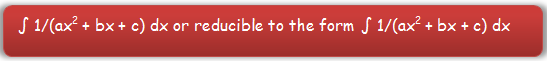In such type of integrals we aim at expressing the term ax2 + bx + c as the sum or difference of two squares. For doing so, we follow the following steps:

(a) Try to make the coefficient of x2 as unity by multiplying or dividing by it throughout (obviously, if it is not unity)

(b) Add and subtract the square of half of the coefficient of x to express it in the form [(x + (b/2a))2 + (4ac-b2)/4a2].

(c) This reduces the given expression into one of the following forms which can be easily integrated using the formulae

∫1/(x2 - a2) or ∫1/(a2 + x2) or ∫1/(a2 - x2)

2. Integrals of the form: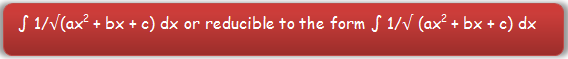For integrating such functions, we follow the following steps:

(a) Try to make the coefficient of x2 as unity (if it is not so).

(b) Add and subtract the square of half of the coefficient of x i.e. (1/2 coefficient of x)2 inside the square root to express the term within the square root in the form [(x + (b/2a))2 + (4ac-b2)/4a2] or (4ac-b2)/4a2] – (x + (b/2a))2.

(c) This again reduces the given expression into one of the following forms which can be easily integrated using the formulae

∫ 1/√(x2 - a2) or ∫ 1/√(a2 + x2) or ∫ 1/√(a2 - x2).

3. Integrals of the form: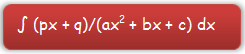(a) In such cases, express the numerator as the sum of constant times differentiation of denominator and a constant, i.e.

Write px + q = A(2ax + b) + C, where 2ax + b = d/dx (ax2 + bx + c) and A and C are arbitrary constants.

(b) The values of the constants can be easily obtained by equating the coefficients of like powers on both the sides.

(c) Replace the numerator (px + q) by A(2ax + b) + C in the given integral which would yield

∫ (px + q)/(ax2 + bx + c) dx =  A ∫ (2ax + b)/(ax2 + bx + c) dx  + C ∫ 1/(ax2 + bx + c) dx

(d) The R.H.S. thus obtained in the previous step can easily be integrated and then the values of the constants as obtained above can be substituted.

4. Integrals of the form: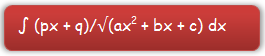In this case also, we proceed on similar lines as explained above in the last case:

(a) Express the numerator as the sum of constant times differentiation of denominator and a constant, i.e.

Write px + q = A(2ax + b) + C, where 2ax + b = d/dx (ax2 + bx + c) and A and C are arbitrary constants.

(b) Try to compute the values of constants by equating the coefficients of like powers of x on both the sides.

(c) Replace the numerator (px + q) by A(2ax + b) + C in the given integral which would yield

∫ (px + q)/ √(ax2 + bx + c) dx =  A ∫ (2ax + b)/√(ax2 + bx + c) dx + C ∫ 1/√(ax2 + bx + c) dx

(d) This R.H.S. can easily be integrated and then the values of the constants as obained above can be substituted.

5. Integrals of the form: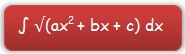In such type of integrals, students are advised to follow the following steps:

(a) Try to make the coefficient of x2 as 1 by dividing by its coefficient (i.e. ‘a’) throughout so as to get x2 + b/a x + c/a.

(b) We again try to make the term a perfect square by adding and subtracting the square of half of coefficient of x and obtain (x + b/2a)2 + (4ac-b2)/4a2.

(c) Henceforth, the integral reduces to one of the following forms

∫√a2+x2 dx, ∫√a2-x2 dx or ∫√x2-a2 dx.

(d) These integrals can be easily computed by applying direct formulae.

6. Integrals of the form: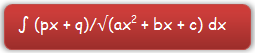(a) In such cases, express the numerator as the sum of constant times differentiation of denominator and a constant, i.e.

Write px + q = C1 (d/dx (ax2 + bx + c) + C2, where  C1 and C2 are arbitrary constants.

(b) The values of the constants can be easily obtained by equating the coefficients of like powers on both the sides.

(c) Replace the numerator (px + q) by C1 (2ax + b) + C2 in the given integral which would yield

∫ (px + q)/√(ax2 + bx + c) dx =  C1 ∫ (2ax + b)/√(ax2 + bx + c) dx  + C2 ∫ 1/√(ax2 + bx + c) dx

(d) The R.H.S. thus obtained in the previous step can easily be integrated and then the values of the constants as obtained above can be substituted.

7. Integrals of the form:(a) Express (px + q) as px + q = A (2ax + b) + B, where A and B are constants.

(b) Now,by equating the coefficients of x and constant terms on both the sides, we can obtain the values of A and B.

(c) By replacing (px + q) in the given integral by A (2ax + b) + B we obtain the following form:

∫ (px + q) √(ax2 + bx + c) dx = A ∫(2ax + b)√(ax2 + bx + c) dx + B ∫√(ax2 + bx + c) dx

(d) These integrals thus obtained can be easily computed by applying direct formulae.

Watch this Video for more reference

Example:

Evaluate.

Solution:

Let x + 1 = (differential coefficient of 2x2 + x – 3) + B

x +1 = A (4x + 1) + B = 4ax + A + B

Hence, equating the coefficients we get, A = 1/4, B = 3/4

Now  I ==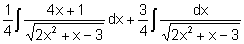Let  I1 =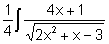and  I2 =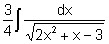Put  2x2 + x – 3 = z ⇒ (4x +1) dx = dz

I1 =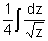=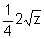=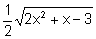I2 =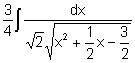==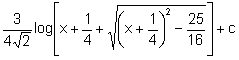Hence  I =.Integration of Irrational Algebraic Fractions

Finding the integrals of irrational functions can often be a cumbersome task and hence if we try to compute them directly following the usual rules, we might fail in reaching at the solutions. Hence, students are advised to remember the below listed substitutions as with their help one can easily get solutions in two-three steps.

1. Irrational functions of (ax + b)1/n  and x can be easily evaluated by the substitution tn = ax + b. Thus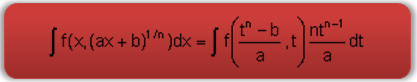2. In the following type of integrals, the substitution x – k = 1/t proves useful.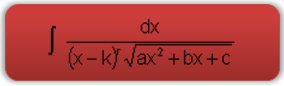With the help of this substitution, the given integral takes the form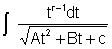which can be easily integrated.

3. Integrals of the form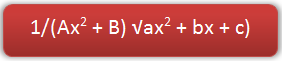can be solved by putting x = 1/t so that the integral reduces to the form: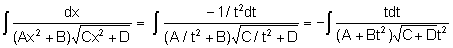.

Now the substitution C + Dt2 = u2 reduces it to the form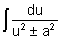.

4. Integrals of the form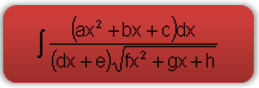Here, we write, ax2 + bx + c  = A1 (dx + e) (2fx + g) + B1(dx + e) + C1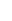where A1, B1 and C1 are constants which can be obtained by comparing the coefficient of like terms on both sides. And given integral will reduce to the form

A1this can be evaluated using the methods discussed above.

Example:

Evaluate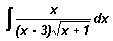Solution:

The given integral isPut x + 1 = t2, we get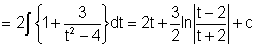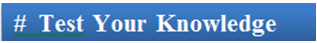Q1. While solving certain type of integrals, we try to convert the coefficient of one of the term as unity. That term is

(a) the coefficient of x2.

(b) the coefficient of x

(c) constant term

(d) none of these

Q2. In integrals of the form ∫(ax + b)/(px2 + qx + r) dx, the substitution used is

(a) express denominator as constant times numerator

(b) numerator as multiple of denominator

(c) ax + b = A(2px + q) + C

(d) none of these

Q3. While solving integrals of the form ∫ (px + q)/√(ax2 + bx + c) dx, express

(a) denominator as sum of constant and differentiation of numerator.

(b) numerator as the sum of constant times differentiation of denominator and a constant

(c) the coefficient of x2 as unity

(d) none of these

Q4. Irrational functions of the form (ax+b)1/n  and x can be easily evaluated by

(a) substituting ax + b = Cn + D.

(b) using partial fractions

(c) equating this term to the sum of a nth power term and a constant.

(d) the substitution tn = ax + b.

Q5. The integrals of the form ∫(ax2 + bx + c) dx can be reduced to one of the following type of integrals

(a) ∫(a2+ x2) dx, ∫(a2 - x2) dx or ∫(x2 - a2) dx

(b) x2/a + bx/a + c

(c) ∫√(a2 + x2) dx, ∫√(a2 - x2) dx or ∫√(x2 - a2) dx

(d) none of theseQ1.

Q2.

Q3.

Q4.

Q5.

(a)

(c)

(b)

(d)

(c)

Related Resources

You may wish to refer indefinite integral.

For getting an idea of the type of questions asked, refer the previous year papers.

To read more, Buy study materials of Indefinite integral comprising study notes, revision notes, video lectures, previous year solved questions etc. Also browse for more study materials on Mathematics here.
```### Course Features

• 731 Video Lectures
• Revision Notes
• Previous Year Papers
• Mind Map
• Study Planner
• NCERT Solutions
• Discussion Forum
• Test paper with Video Solution